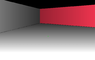# I can't figure out how to reverse my function

• I
• newjerseyrunner

#### newjerseyrunner

I have this function that I need to reverse.

int getYPosition(float height, float dist) const {
const float hh = renderer -> viewPortHalfH / dist;
return renderer -> viewPortHalfH - hh + hh * ((height + renderer -> player -> verticalAngle * dist) / 128.0f);
}

float getDistYPosition(float height, int yPosition) const {
//wtf, I can't figure out what this should be
return 1;
}

I can't figure out how to reverse it though, I should be able to take the resulting int, put it through a function with the height value and get back distance. Normally, when I get stuck, I just ask wolfram alpha to solve it for me, but that doesn't seem to be giving me an answer.

y = k - (k/d) + (k/d) * ((h + v * d) / 128) solve d
Is there something with my equation that makes it fundamentally irreversible?

I feel like wolfram alpha is having trouble because the general equation has some quirks that the actual code won't? Like the fact that both renderer -> viewPortHalfH and dist are always positive and non-zero. How would I tell wolfram alpha about this (would it even help?)

Are you trying to implement the Pythagorean distance here?

y = k - (k/d) + (k/d) * ((h + v * d) / 128)
Multiply the equation by d and you get a standard linear equation which you can solve for d.

•newjerseyrunner
Are you trying to implement the Pythagorean distance here?
No. I'm not sure what it's called. The first function projects a 2.5D point to a 2D screen, I'm trying to reverse the projection. I don't know x or y position, in fact, I need this distance calculation to figure that out in order to texture it.It's for determining distance from a camera across the floor. I knew the distance of the bottom of the wall, and I knew what pixel height it started at. I then need to iterate along each pixel going down and figure out it's distance from the camera.

Multiply the equation by d and you get a standard linear equation which you can solve for d.
That was the step I needed, I was able to figure out the algorithm from there

float yPositionToDist(float height, int pixel) const {
float top = (height - 128.0f) * renderer -> viewPortHalfH;
float bottom = (renderer -> player -> verticalAngle + 128.0f) * renderer -> viewPortHalfH - 128.0f * (float)pixel;
return -top / bottom;

•jedishrfu